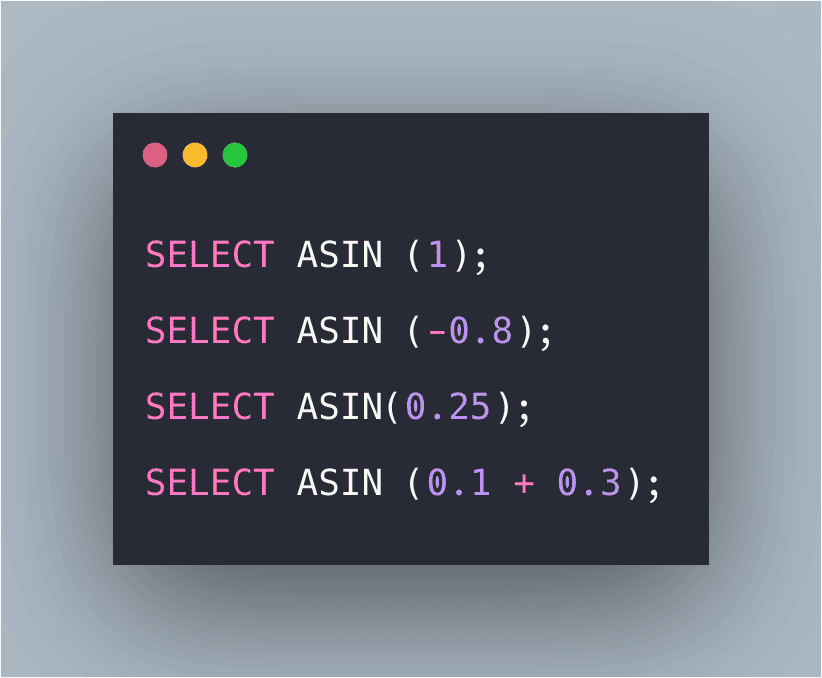# SQL ASIN() Function: The Complete Guide

0
1SQL ASIN() function returns the arc sine of a number. The specified number must be between -1 to 1; otherwise, this function returns NULL.

## SQL ASIN()

SQL ASIN() is a built-in function that is used for finding the arc sine of a number. The number should be in the range of -1 to 1; otherwise, it will return NULL.

### Syntax

```SELECT ASIN(Expression);
```

### Parameters

Expression: The Expression whose cosine value is to be retrieved.

### Examples

#### Query 1

```SELECT ASIN (1);
```

#### Output

`1.5707963267949`

#### Explanation

Here, the arcsine value is returned.

#### Query 2

`SELECT ASIN (-0.8);`

#### Output

```-0.9272952180016123
```

#### Explanation

Here, the arcsine value is returned.

#### Query 3

```SELECT ASIN (NULL);
```

`NULL`

#### Explanation

Passing of NULL value as an argument will return a NULL value.

#### Query 4

```SELECT ASIN(0.25);
```

#### Output

```0.25268025514207865
```

#### Explanation

Here, the arcsine value is returned, which is of fractional type.

#### Query 5

```SELECT ASIN (0.1 + 0.3);
```

#### Output

`0.411516846067488`

#### Explanation

Here, the arcsine value of the expressions is returned.

## Conclusion

SQL Server ASIN() function returns the arcsine of a number.

That’s it for this tutorial.